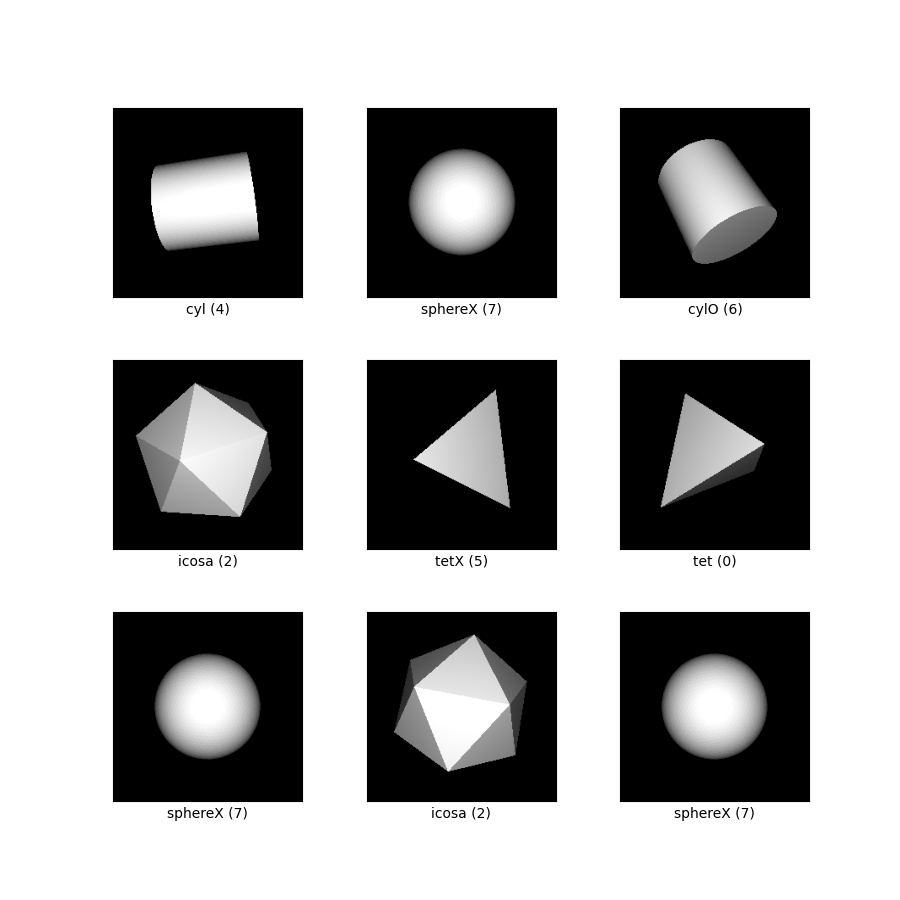Tune in to the first Women in ML Symposium this Tuesday, October 19 at 9am PST

# symmetric_solids

• Description:

This is a pose estimation dataset, consisting of symmetric 3D shapes where multiple orientations are visually indistinguishable. The challenge is to predict all equivalent orientations when only one orientation is paired with each image during training (as is the scenario for most pose estimation datasets). In contrast to most pose estimation datasets, the full set of equivalent orientations is available for evaluation.

There are eight shapes total, each rendered from 50,000 viewpoints distributed uniformly at random over the full space of 3D rotations. Five of the shapes are featureless -- tetrahedron, cube, icosahedron, cone, and cylinder. Of those, the three Platonic solids (tetrahedron, cube, icosahedron) are annotated with their 12-, 24-, and 60-fold discrete symmetries, respectively. The cone and cylinder are annotated with their continuous symmetries discretized at 1 degree intervals. These symmetries are provided for evaluation; the intended supervision is only a single rotation with each image.

The remaining three shapes are marked with a distinguishing feature. There is a tetrahedron with one red-colored face, a cylinder with an off-center dot, and a sphere with an X capped by a dot. Whether or not the distinguishing feature is visible, the space of possible orientations is reduced. We do not provide the set of equivalent rotations for these shapes.

Each example contains of

• the 224x224 RGB image
• a shape index so that the dataset may be filtered by shape.
The indices correspond to:

• 0 = tetrahedron
• 1 = cube
• 2 = icosahedron
• 3 = cone
• 4 = cylinder
• 5 = marked tetrahedron
• 6 = marked cylinder
• 7 = marked sphere
• the rotation used in the rendering process, represented as a 3x3 rotation matrix

• the set of known equivalent rotations under symmetry, for evaluation.

In the case of the three marked shapes, this is only the rendering rotation.

• Homepage: https://implicit-pdf.github.io

• Versions:

• `1.0.0` (default): Initial release.
• Download size: `3.10 GiB`

• Dataset size: `3.94 GiB`

• Auto-cached (documentation): No

• Splits:

Split Examples
`'test'` 40,000
`'train'` 360,000
• Features:
``````FeaturesDict({
'image': Image(shape=(224, 224, 3), dtype=tf.uint8),
'label_shape': ClassLabel(shape=(), dtype=tf.int64, num_classes=8),
'rotation': Tensor(shape=(3, 3), dtype=tf.float32),
'rotations_equivalent': Tensor(shape=(None, 3, 3), dtype=tf.float32),
})
``````• Citation:
``````@inproceedings{implicitpdf2021,
title = {Implicit Representation of Probability Distributions on the Rotation
Manifold},
author = {Murphy, Kieran and Esteves, Carlos and Jampani, Varun and